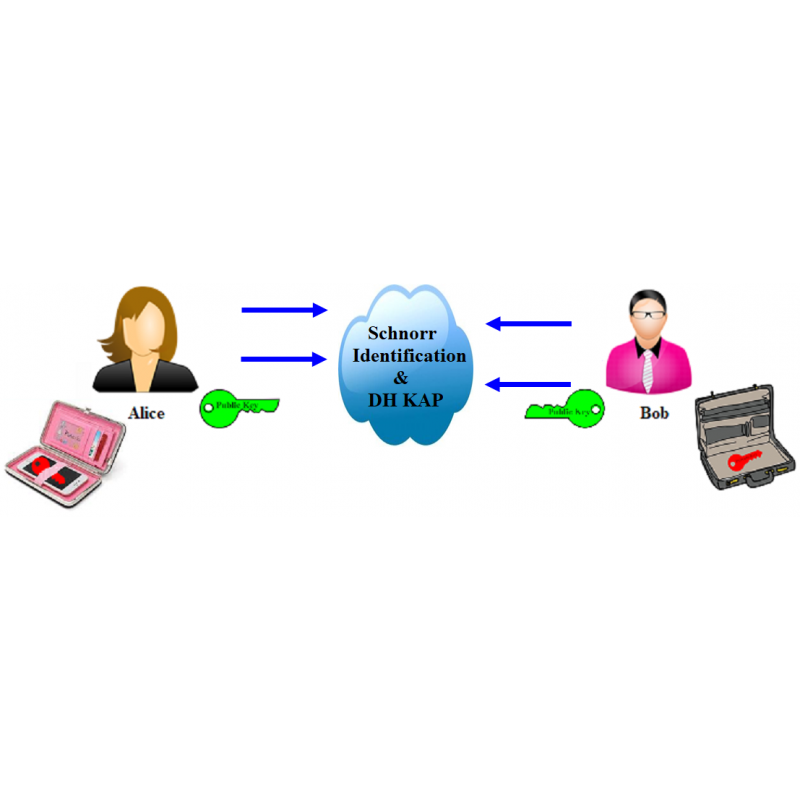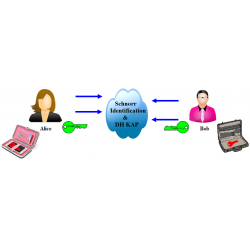•# Authenticated KAP with RSA

€4.00
Tax included

Authenticated Key Agreement Protocol (KAP) with RSA signature

Quantity

This protocol is executed between Student Alice (You) and Mentor Bob.

Parameter values sent by Alice are included in brackets [ ] and must NOT be entered in the corresponding input fields.

The following functions are used in the protocol:

>>mod_exp(g,x,p)

>>randi(z)

>>genprime(nob)

>>genstrongprime(nob)

>>eeuklid(e,φ)

1. Generate two prime numbers p and q having length less than 20 bits using function genprime(). Send numbers [p,q] for verification to the Mentor.

2. Calculate value n=p*q and Euler's totient function's value φ=(p-1)*(q-1). Send [n,φ] for verification to the Mentor.

3. Choose RSA exponent e satisfying condition gcd(e, φ)=1 and private key d using extended Euclidean algorithm. Send [e,d] for verification to the Mentor.

4. Generate prime number p', private key x, where 1 < x < p' - 1 and find generator g. Send [p',g,x] for verification to the Mentor.

5. Find your public key PuK_A = g^x mod p' and sign it with RSA parameters. Send  [PuK_A,s_A] for verification to the Mentor.

6. Mentor sent his parameters (PuK_B,s_B,e_m,n_m). Verify signature and form key pair K_ab. Send [K_ab] for verification to the Mentor.

3152 Items